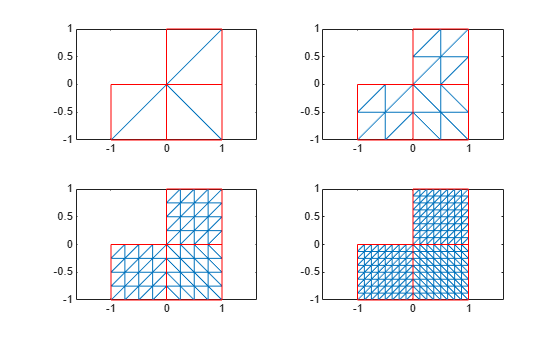Documentation

# refinemesh

Refine triangular mesh

This page describes the legacy workflow. New features might not be compatible with the legacy workflow. For the corresponding step in the recommended workflow, see `generateMesh`.

## Syntax

```[p1,e1,t1] = refinemesh(g,p,e,t)
[p1,e1,t1] = refinemesh(g,p,e,t,'regular')
[p1,e1,t1] = refinemesh(g,p,e,t,'longest')
[p1,e1,t1] = refinemesh(g,p,e,t,it)
[p1,e1,t1] = refinemesh(g,p,e,t,it,'regular')
[p1,e1,t1] = refinemesh(g,p,e,t,it,'longest')
[p1,e1,t1,u1] = refinemesh(g,p,e,t,u)
[p1,e1,t1,u1] = refinemesh(g,p,e,t,u,'regular')
[p1,e1,t1,u1] = refinemesh(g,p,e,t,u,'longest')
[p1,e1,t1,u1] = refinemesh(g,p,e,t,u,it)
[p1,e1,t1,u1] = refinemesh(g,p,e,t,u,it,'regular')
[p1,e1,t1,u1] = refinemesh(g,p,e,t,u,it,'longest')
```

## Description

`[p1,e1,t1] = refinemesh(g,p,e,t)` returns a refined version of the triangular mesh specified by the geometry `g`, Point matrix `p`, Edge matrix `e`, and Triangle matrix `t`.

The triangular mesh is given by the mesh data `p`, `e`, and `t`. For details on the mesh data representation, see Mesh Data.

`[p1,e1,t1,u1] = refinemesh(g,p,e,t,u)` refines the mesh and also extends the function `u` to the new mesh by linear interpolation. The number of rows in `u` should correspond to the number of columns in `p`, and `u1` has as many rows as there are points in `p1`. Each column of `u` is interpolated separately.

An extra input argument `it` is interpreted as a list of subdomains to refine, if it is a row vector, or a list of triangles to refine, if it is a column vector.

The default refinement method is regular refinement, where all of the specified triangles are divided into four triangles of the same shape. Longest edge refinement, where the longest edge of each specified triangle is bisected, can be demanded by giving `longest` as a final parameter. Using `regular` as a final parameter results in regular refinement. Some triangles outside of the specified set may also be refined to preserve the triangulation and its quality.

## Examples

collapse all

Refine the mesh of the L-shaped membrane several times. Plot the mesh for the geometry of the L-shaped membrane.

```[p,e,t] = initmesh('lshapeg','hmax',inf); subplot(2,2,1), pdemesh(p,e,t) [p,e,t] = refinemesh('lshapeg',p,e,t); subplot(2,2,2), pdemesh(p,e,t) [p,e,t] = refinemesh('lshapeg',p,e,t); subplot(2,2,3), pdemesh(p,e,t) [p,e,t] = refinemesh('lshapeg',p,e,t); subplot(2,2,4), pdemesh(p,e,t) ````subplot`

## Algorithms

The algorithm is described by the following steps:

1. Pick the initial set of triangles to be refined.

2. Either divide all edges of the selected triangles in half (regular refinement), or divide the longest edge in half (longest edge refinement).

3. Divide the longest edge of any triangle that has a divided edge.

4. Repeat step 3 until no further edges are divided.

5. Introduce new points of all divided edges, and replace all divided entries in `e` by two new entries.

6. Form the new triangles. If all three sides are divided, new triangles are formed by joining the side midpoints. If two sides are divided, the midpoint of the longest edge is joined with the opposing corner and with the other midpoint. If only the longest edge is divided, its midpoint is joined with the opposing corner.